## RS Aggarwal Class 6 Solutions Chapter 14 Constructions (Using Ruler and a Pairs of Compasses) Ex 14A

These Solutions are part of RS Aggarwal Solutions Class 6. Here we have given RS Aggarwal Solutions Class 6 Chapter 14 Constructions (Using Ruler and a Pairs of Compasses) Ex 14A.

Other Exercises

Question 1.
Solution:
Steps of construction :
(i) Draw a line segment PQ = 6.2 cm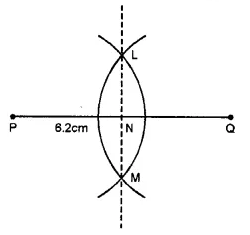(ii) With centre P and Q and radius more than half of PQ, draw arcs on each side intersecting each other at L and M.
(iii) Join LM intersecting PQ at N.
Then, LM is the perpendicular bisector of PQ.

Question 2.
Solution:
Steps of Construction :
1. Draw a line segment AB = 5.6 cm.
2. With A as centre and radius more than half AB, draw arcs, one one each side of AB.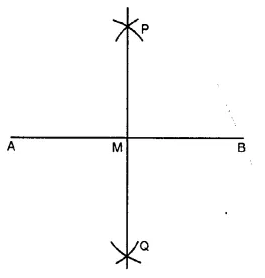3. With B as centre and same radius as before, draw arcs, cutting the previous arcs at P and Q respectively.
4. Join P and Q, meeting AB at M. Then PQ is the required perpendicular bisector of AB.
Verification : Measure ∠AMP. We see that ∠AMP = 90°. So, PQ is the perpendicular bisector of AB.

Question 3.
Solution:
Steps of Contruction :
1. Draw a ray RX.
2. With O as centre and any radius draw an arc cutting OA and OB at P and Q respectively.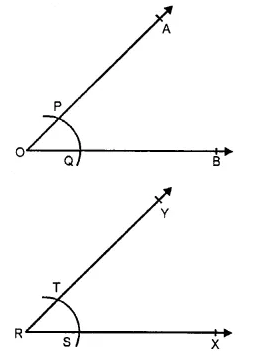3. With R as centre and same radius draw an arc cutting RX at S.
4. With S as centre and radius PQ cut the arc through S at T.
5. Join RT and produce it to Y. Then ∠XRY is the required angle equal to ∠AOB.
Verification: Measuring angle AOB and ∠XRY, we observe that ∠XRY = ∠AOB.

Question 4.
Solution:
Steps of constructions :
(i) Draw an angle ABC = 50° with the help of a protractor.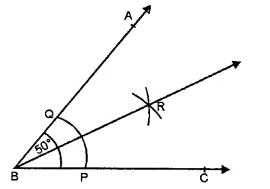(ii) With centre B and C and a suitable radius, draw an arc meeting AB at Q and BC at P.
(iii) With centres P and Q and with a suitable radius draw two arcs intersecting each other at R inside the angle ABC.
(iv) JoinRB.
Then ray BR is the bisector of ∠ABC.

Question 5.
Solution:
Steps of construction :
(i) Draw an angle AOB = 85° with the help of the protractor.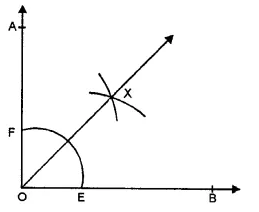(ii) With centre O, draw an arc with a suitable radius meeting OB at E and OA at F.
(iii) With centre E and F and with a suitable radius draw arcs intersecting each other at X inside the angle AOB.
Then ray OX is the bisector of ∠AOB.

Question 6.
Solution:
Steps of Construction :
(1) Draw the given line AB and take a point P on it.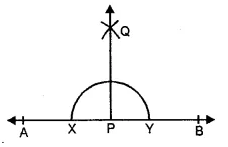(2) With P as centre and any suitable radius draw a semi-circle to cut the line AB at X and Y.
(3) With centre X and radius more than XP draw an arc.
(4) With centre Y and same radius draw another arc to cut the previous arc at
(5) Join PQ. Then, PQ is the required line passing through P and perpendicular to AB.
Verification : Measure ∠APQ, we see that ∠APQ = 90°

Question 7.
Solution:
Steps of Construction :
(1) Draw the given line AB and take a point P outside it.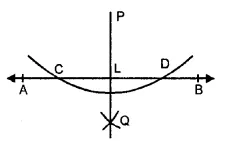(2) With P as centre and suitable radius, draw an arc intersecting AB at C and D.
(3) With C as centre and radius more than half CD, draw an arc.
(4) With D as centre and same radius, draw another arc to cut the previous arc at Q.
(5) Join PQ, meeting AB at L. Then PL is the required line passing through P and perpendicular to AB.
Verification : Measure ∠PLB. We see that ∠PLB = 90°.

Question 8.
Solution:
Steps of Construction :
1. Draw a given line AB and take a point P outside it.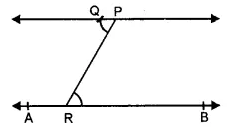2. Take a point R on AB
3. Join PR.
4. Draw ∠RPQ such that ∠RPQ = ∠PRB as shown in the figure.
5. Produce PQ on both sides to form a line. Then, PQ is the required line passing through P and parallel to AB.
Verification: Since ∠RPQ = ∠PRB and these are alternate interior angles, it follows that PQ || AB.

Question 9.
Solution:
Steps of Construction :
1. Draw a ray BX and cut of BC = 5 cm.
2. With B as centre and suitable radius draw an arc above BX and cutting it at P.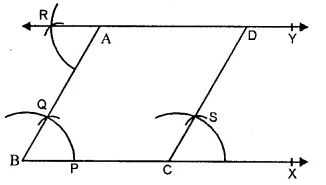3. With P as centre and the same radius as before draw another arc to cut the previous arc at Q.
4. Join PQ and produce it to the point A such that. AB = 4.5 cm. Then ∠ABC = 60° is the required angle.
5. Draw ∆RAB such that ∆RAB = ∆ABC.
6. Produce RA on both sides to form a line. Then, RY is the line parallel to BC and passing through A.
7. Now, draw ∆SCX = ∆ABC at the point C.
8. Produce CS to intersect the line RY at D.Then CD is the required line through C and parallel to AB.
9. Measure AB and CD. We see that AD = 5 cm. and CD = 4.5 cm.
Verification. Since ∠RAB = ∠ABC and these are alternate angles, it follows that RY || BC.
Also ∠SCX = ∠ABC and these are corresponding angles, it follows that CD || AB.

Question 10.
Solution:
Steps of Construction :
1. With the help of a rular, draw a line segment AB = 6 cm. and off AC = 2.5 cm such that the point C is on AB.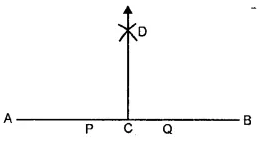2. With C as centre and any suitable radius draw a semi-circle to cut AB at P and
3. With P as centre and any radius more than PC draw an arc.
4. With Q as centre and same radius draw another arc to cut the previous arc at D.
5. Join CD. Then CD is the required line perpendicular to AB.
Verification : Measure ∠ACD. We see that ∠ACD = 90°.

Question 11.
Solution:
Steps of Construction :
1. With the help of rular, draw a line segment AB = 5.6 cm.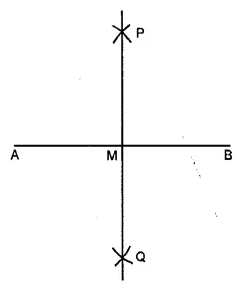2. With A as centre and radius more than half AB, draw arcs, one on each side of AB.
3. With B as centre and the same radius as before draw arcs, cutting the previous arcs at P and Q respectively.
4. Join PQ, meeting AB at M. Then, PQ is the required right bisector of AB.
Verification : On measuring AM and BM and ∠AMP, we see that AM = BM and ∠AMP = 90°.
So, PQ is the right bisector of AB.

Question 12.
Solution:
Steps of Construction :
1. With the help of a rular, draw a ray OA.
2. With O as centre and suitable radius draw an arc to cut OA at P.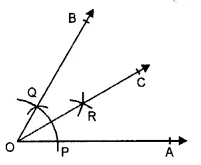3. With P as centre and the same radius, draw another are to cut the previous arc at Q.
4. Join OQ and produce it to any point B, then ∠AOB = 60° is the required angle.
5. With P as centre and radius more than half PQ, draw an arc.
6. With Q as centre and the same radius, draw another arc to cut the previous arc at R.
7. Join OR and produce it to the point C. Then OC is the required bisector of ∠AOB.
Verification : Measure ∠AOC and ∠BOC. We see that ∠AOC = ∠BOC. So, OC is the bisector of ∠AOB.

Question 13.
Solution:
Steps of construction :
1. Draw a ray OA with the help of a rular.
2. With O as centre and suitable radius draw an arc above OA to cut it at P.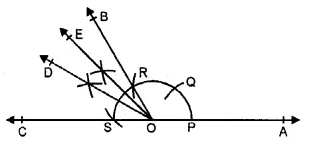3. With P as centre and same radius, cut the arc at Q and again with Q as centre and same radius cut the arc at R. With R as centre and same radius, again cut the arc at S.
4. Join OR and produce it to B and join OS and produce it to C.
5. Draw the bisector OD of ∠BOC.
6. Draw the bisector OE of ∠BOD. Then, ∠AOE = 135° is the required angle.

Hope given RS Aggarwal Solutions Class 6 Chapter 14 Constructions Ex 14A are helpful to complete your math homework.

If you have any doubts, please comment below. Learn Insta try to provide online math tutoring for you.

## RS Aggarwal Class 6 Solutions Chapter 13 Angles and Their Measurement Ex 13D

These Solutions are part of RS Aggarwal Solutions Class 6. Here we have given RS Aggarwal Solutions Class 6 Chapter 13 Angles and Their Measurement Ex 13D.

Other Exercises

Objective questions
Mark against the correct answer in each of following.

Question 1.
Solution:
(c) vertex of an angle lie on it.

Question 2.
Solution:
(c) an angle.

Question 3.
Solution:
(c) A straight angle has 180°

Question 4.
Solution:
An angle measuring 90° is called a right angle. (b)

Question 5.
Solution:
An angle measuring 91° is an obtuse angle as it is more than 90° and less than 180°.(b)

Question 6.
Solution:
An angle measuring 270° is a reflex angles as it is greater than 180° and less than 360°. (d)

Question 7.
Solution:
(c) A straight angle is equal to 180°

Question 8.
Solution:
(c) A reflex angles is greater than 180° but less than 360°

Question 9.
Solution:
(d) A complete angle is equal to 360°.

Question 10.
Solution:
(b) A reflex angle is greater than 180° but less than 360°.

Question 11.
Solution:
Two right angles = (2 x 90)°
= 180° (b)

Question 12.
Solution:
$$\frac { 3 }{ 2 }$$ of a right angle = $$\frac { 3 }{ 2 }$$ x 90° = 135° as 1 right angle = 90° (b)

Question 13.
Solution:
36 spokes has 360°
= $$\frac { { 360 }^{ O } }{ { 36 }^{ O } }$$ = 10° (c)

Hope given RS Aggarwal Solutions Class 6 Chapter 13 Angles and Their Measurement Ex 13D are helpful to complete your math homework.

If you have any doubts, please comment below. Learn Insta try to provide online math tutoring for you.

## RS Aggarwal Class 6 Solutions Chapter 13 Angles and Their Measurement Ex 13C

These Solutions are part of RS Aggarwal Solutions Class 6. Here we have given RS Aggarwal Solutions Class 6 Chapter 13 Angles and Their Measurement Ex 13C.

Other Exercises

Question 1.
Solution:
(i) Place the protractor in such a way that its centre is exactly at the vertex O of the given angle AOB and the base line lies along the arm OA. Read off the mark through which the arm OB passes, starting from 0° on the side A.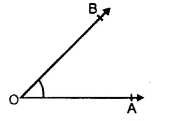We find that ∠AOB = 45°.
(ii) The given angle is ∠PQR. Place the protractor in such a way that its centre is exactly on the vertex Q of the given angle and the base line lies along the arm QR.Read off the mark through which the arm QP passes, starting from 0° on the side of R.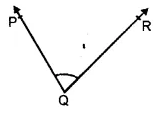We find that ∠PQR = 67°
(in) The given angle is ∠DEF. Place the protractor in such a way that its centre is exactly on the vertex E of the given angle and the base line lies along the arm ED. Read off the mark through which the arm EF passes, starting from 0° on the side of D.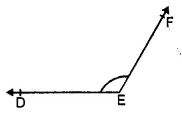We find that ∠DEF = 130°
(iv) The given angle is ∠LMN. Place the protractor in such a way that its centre is exactly on the vertex M of the given angle and the base line lies along the arm ML. Read off the mark through which the arm MN passes, starting from 0° on the side of L.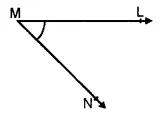We find that ∠LMN = 50°
(v) The given angle is ∠RST. Place the protractor in such a way that its centre is exactly on the vertex S of the given angle and the base line lies along the arm SR. Read off the mark through which the arm ST passes, starting from 0° on the side of R.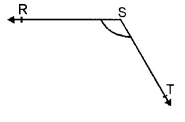We find that the ∠RST = 130°.
(vi) The given angle is ∠GHI. Place the protractor in such a way that its centre is exactly on the vertex H of the given angle and the base line lies along the arm HI. Read off the mark through which the arm HG passes, starting from 0° on the side of I.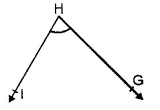We find that ∠GHI = 70°

Question 2.
Solution:
(i) Draw a ray OA. Place the protractor in such a way that its centre lies exactly at O and the base line lies along OA. Starting from 0° on the side of A, look for the 25° mark on the protractor. Mark a point B at this 25° mark. Remove the protractor and draw the ray OB. Then ∠AOB is the required angle.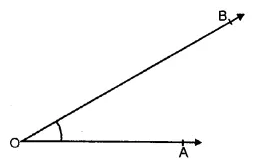(ii) Draw a ray OA. Place the protractor in such a way that its centre lies exactly at O and the base line lies along OA. Starting from 0° on the side of A, look for the 72° mark on the protractor. Mark a point B at this 72° mark. Remove the protractor and draw the ray OB. Then,
∠AOB is the required angle of measure 72°.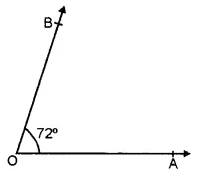(iii) Draw a ray OA. Place the protractor in such a way that its centre lies exactly at O and the base line lies along OA. Starting from 0° on the side of A, look for the 90° mark on the protractor. Mark a point B at this 90° mark. Remove the protractor and draw the ray OB. Then ∠AOB is the required angle whose measure is 90°.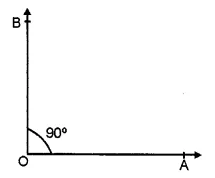(iv) Draw a ray OA. Place the protractor in such a way that its centre exactly lies at O and the base line lies along OA. Starting from 0° on the side of A, look for the 117° mark on the protractor. Mark a point B at this 117° mark. Remove the protractor and draw the ray OB. Then ∠AOB is the required angle whose measure is 117°.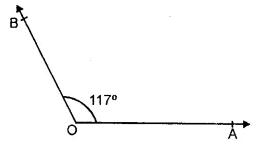(v) Draw a ray OP. Place the protractor in such a way that its centre lies exactly at O and the base line lies along OP. Starting from 0° on the side of P, look for the 165° mark on the protractor. Mark a point Q at this 165° mark. Remove the protractor and draw the ray OQ. Then, ∠POQ is the required angle whose measure is 165°.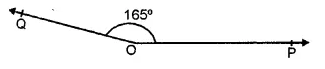(vi) Draw a ray OP. Place the protractor in such a way that its centre lies exactly at O and the base line lies along OP. Starting from 0° on the side of P, look for the 23° mark on the protractor. Mark a point Q at this 23° mark. Remove the protractor and draw the ray OQ. Then ∠POQ is the required angle whose measure is 23°.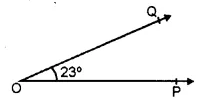(vii) Draw a ray OA. Place the protractor in such a way that its centre lies exactly at O and the base line lies along OA. Starting from 0° on the side of A,Took for the 180° mark on the protractor. Mark a point B on this 180° mark. Remove the protractor and draw the ray OB. Then ∠AOB is the required angle whose measure is 180°.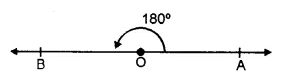(viii) Draw a ray Rs. Place the protractor in such away that its centre lies exactly at R and the base line lies along RS. Starting from 0° on the side of S, look for the 48° mark on the protractor. Mark a point T at this 48° mark. Remove the protractor and draw the ray RT. Then, ∠SRT is the required angle whose measure is 48°.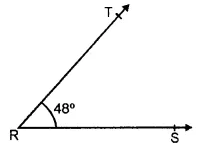Question 3.
Solution:
On measuring the given angle ABC with the help of a protractor, it is 50°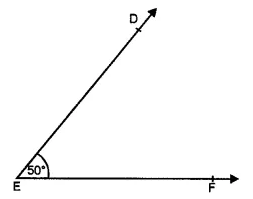Now, place the protractor on EF in such a way that its centre lies on E exactly and base with the line EF.
Now read off the mark through with the arm ED passes at 50°.
Join DE,
Then ∠DEF is equal to 50° i.e. equal to ∠ABC.

Question 4.
Solution:
Steps of construction :
(i) Draw a line segment AB = 6 cm.
(ii) Take a point C on AB such that AC = 4 cm.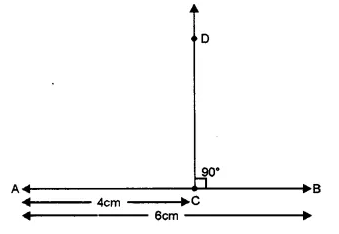(iii) Place protractor with its centre at C and base along CB.
(iv) Mark a point D against 90°.
(v) Remove the protractor and join DC. Then DC ⊥ AB. Ans.

Hope given RS Aggarwal Solutions Class 6 Chapter 13 Angles and Their Measurement Ex 13C are helpful to complete your math homework.

If you have any doubts, please comment below. Learn Insta try to provide online math tutoring for you.

## RS Aggarwal Class 6 Solutions Chapter 13 Angles and Their Measurement Ex 13B

These Solutions are part of RS Aggarwal Solutions Class 6. Here we have given RS Aggarwal Solutions Class 6 Chapter 13 Angles and Their Measurement Ex 13B.

Other Exercises

Question 1.
Solution:
(i) Obtuse angle
(ii) Right angle
(iii) straight angle
(iv) Reflex angle
(v) Acute angle
(vi) Complete angle

Question 2.
Solution:
We know that an acute angle is less than 90°
(ii) a right angle is equal to 90°
(iii) an obtuse angle is greater than 90° but less than 180°
(iv) an angle equal to 180° is a straight angle
(v) angle greater than 180° but less than 360° is called a reflex angle
(vi) angle equal to 360° is called a complete angle and angle equal to 0° is called a zero angle. Now the angles are :
(i) acute
(ii) obtuse
(iii) obtuse
(iv) right
(v) reflex
(vi) complete
(vii) obtuse
(viii) obtuse
(ix) acute
(x) acute
(xi) zero
(xii) acute Ans.

Question 3.
Solution:
(i) One right angle = 90°
(ii) Two right angles = (2 x 90)° = 180°
(iii) Three right angles = (3 x 90)° = 270°
(iv) Four right angles = (4 x 90)° = 360°
(v) $$\frac { 2 }{ 3 }$$ right angle = $$\left( \frac { 2 }{ 3 } \times { 90 }^{ O } \right)$$ = 60°
(vi) 1½ right angle = $$\left( 1\frac { 1 }{ 2 } \times { 90 }^{ O } \right)$$
$$\left( \frac { 3 }{ 2 } \times { 90 }^{ O } \right)$$ = 135°

Question 4.
Solution:
(i) When it is 3 o’ clock, the minute hand is at 12, and hour hand is at 3 as shown in the figure, clearly, the angle between the two hands 90°.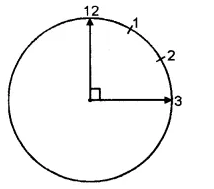(ii) When it is 6 o’ clock, the minute hand is at 12 and the hour hand is at 6 as shown in the figure. Clearly, the angle between the two hands of the clock is a straight angle is i.e. 180°.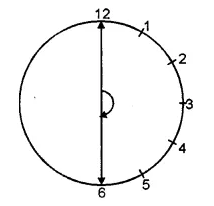(iii) When it is 12 o’ clock, both the hands of the clock lie at 12 as shown in the figure. Clearly, the angle between the two hands = 0°.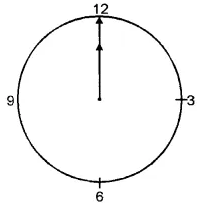(iv) When it is 9 o’ clock, the minute hand is at 12 and the hour hand is at 9 as shown in the figure. Clearly, the angle between the two hands = 90°.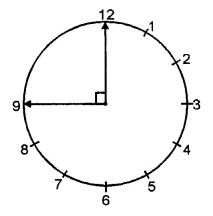Question 5.
Solution:
(i) Take the rular and draw any ray OA. Again using the rular, starting from O, draw a ray OB in such a way that the angle formed is less than 90°. Then, ∠AOB is the required acute angle.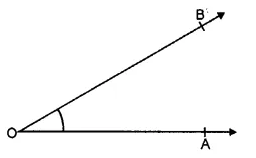(ii) Take the rular and draw any ray OA. Now, starting from O, draw another ray OB, with the help of the rular, such that the angle formed is greater than a right angle.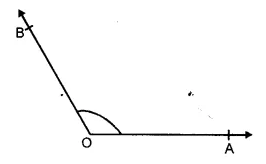Then, ∠AOB is the required obtuse angle.
(iii) Take a rular and draw any ray OA. Now, starting from O, draw ray OB in the opposite direction of the ray OA. Then ∠AOB is the required straight angle.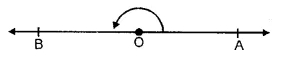Hope given RS Aggarwal Solutions Class 6 Chapter 13 Angles and Their Measurement Ex 13B are helpful to complete your math homework.

If you have any doubts, please comment below. Learn Insta try to provide online math tutoring for you.

## RS Aggarwal Class 6 Solutions Chapter 13 Angles and Their Measurement Ex 13A

These Solutions are part of RS Aggarwal Solutions Class 6. Here we have given RS Aggarwal Solutions Class 6 Chapter 13 Angles and Their Measurement Ex 13A.

Other Exercises

Question 1.
Solution:
Three examples are : Tongs, Scissors and Compasses.

Question 2.
Solution:
In the given angle ABC, the vertex is B and arms are $$\overrightarrow { AB }$$ and $$\overrightarrow { BC }$$ .

Question 3.
Solution:
(i) In the given figure, three angles are formed.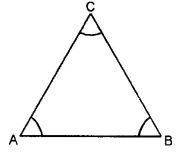Names of the angles are :
∠ABC, ∠BAC and ∠ACB
(ii) In the given figure, four angles are formed.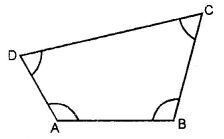(iii) In the given figure, eight angles are formed.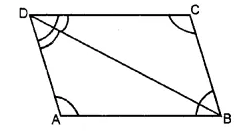Names of the angles are :

Question 4.
Solution:
In given figure
(i) Points S and Q are in the interior of ∠AOB
(ii) Points P and R are in the exterior of ∠AOB.
(iii) Points A, O, B, N, T lie on ∠AOB.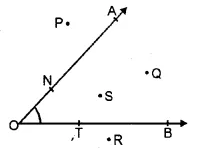Question 5.
Solution:
(i) False
(ii) True
(iii) False
(iv) True
(v) False

Question 6.
Solution:
In the given figure, another name for :
(i) ∠1 is ∠EPB
(ii) ∠2 is ∠PQC
(iii) ∠3 is FQD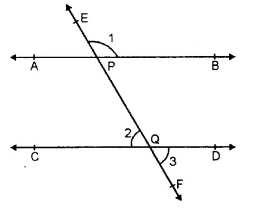Hope given RS Aggarwal Solutions Class 6 Chapter 13 Angles and Their Measurement Ex 13A are helpful to complete your math homework.

If you have any doubts, please comment below. Learn Insta try to provide online math tutoring for you.